Next: Usage Up: Compton Process Previous: Compton Process

### Formulas

In the following,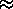is an approximation in the frame where the initial electron and laser collide head-on and the electron is ultra-relativistic.

p,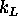,p',k
4-momenta of initial electron, laser photon, final electron and emitted photon, respectively.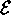,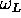,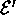,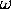Energies of initial electron, laser photon, final electron and emitted photon, respectively.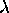Laser energy parameter: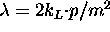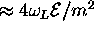.
n
Number of absorbed laser photon. The kinetic relation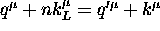holds exactly. Here, q is defined as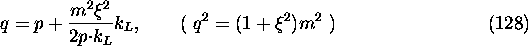(p is replaced by p' for q') and is called quasimomentum.
x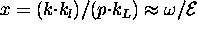, (0<x<1)
v
v=x/(1-x), x=v/(1+v). (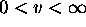)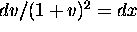.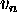Maximum v for given n: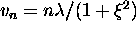.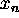Maximum x for given n: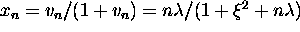.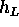Laser helicity (-1 or +1)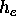,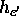Initial and final electron helicities (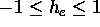)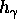Final photon helicity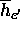,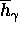`Detector helicity' of the final particles. See section 65 of .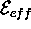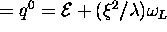is the effective energy of initial electron in the laser field.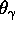Final photon angle.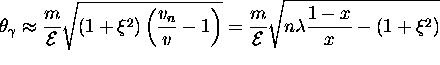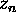The argument of the Bessel functions in the following expressions: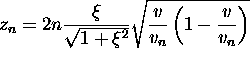Number of photons per unit time is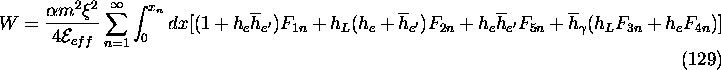The terms involvingandsimultaneously are ignored, i.e., the correlation of polarization between final particles is ignored. The ultra-relativistic approximation has been applied in the terms related to electron helicity (and/or). (Note that the electron helicity is a Lorentz invariant quantity only in the ultra-relativistic limit.)

The sum over the final electron and photon helicities gives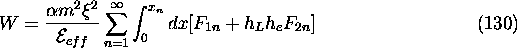The functions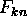are defined by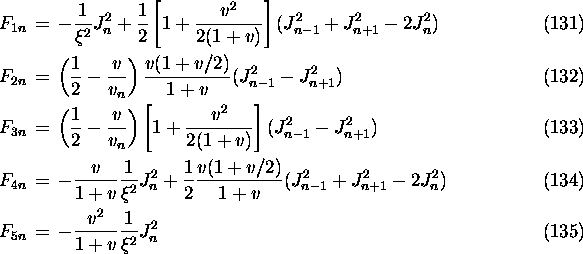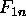,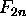,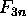,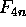are identical to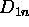,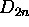,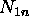,divided by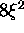in Tsai's paper, although the expressions in his paper look much more complicated.

Once x and n are given, the final momentuma are given, in any frame, by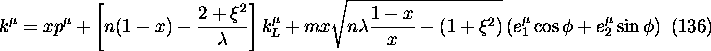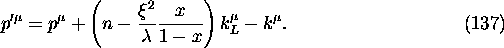Here,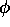is the azimuthal angle in a head-on frame (therefore its distribution is uniform in [0,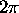]) and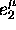and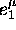are given by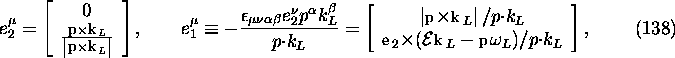where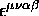is the completely anti-symmetric tensor (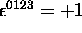). These vectors satisfy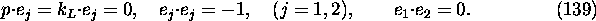The vectorin eq.(139) is ill-defined when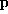and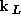are colinear in the original frame. In such a case the spatial part ofis an arbitrary unit vector perpendicular to.Next: Usage Up: Compton Process Previous: Compton Process

Toshiaki Tauchi
Thu Dec 3 17:27:26 JST 1998